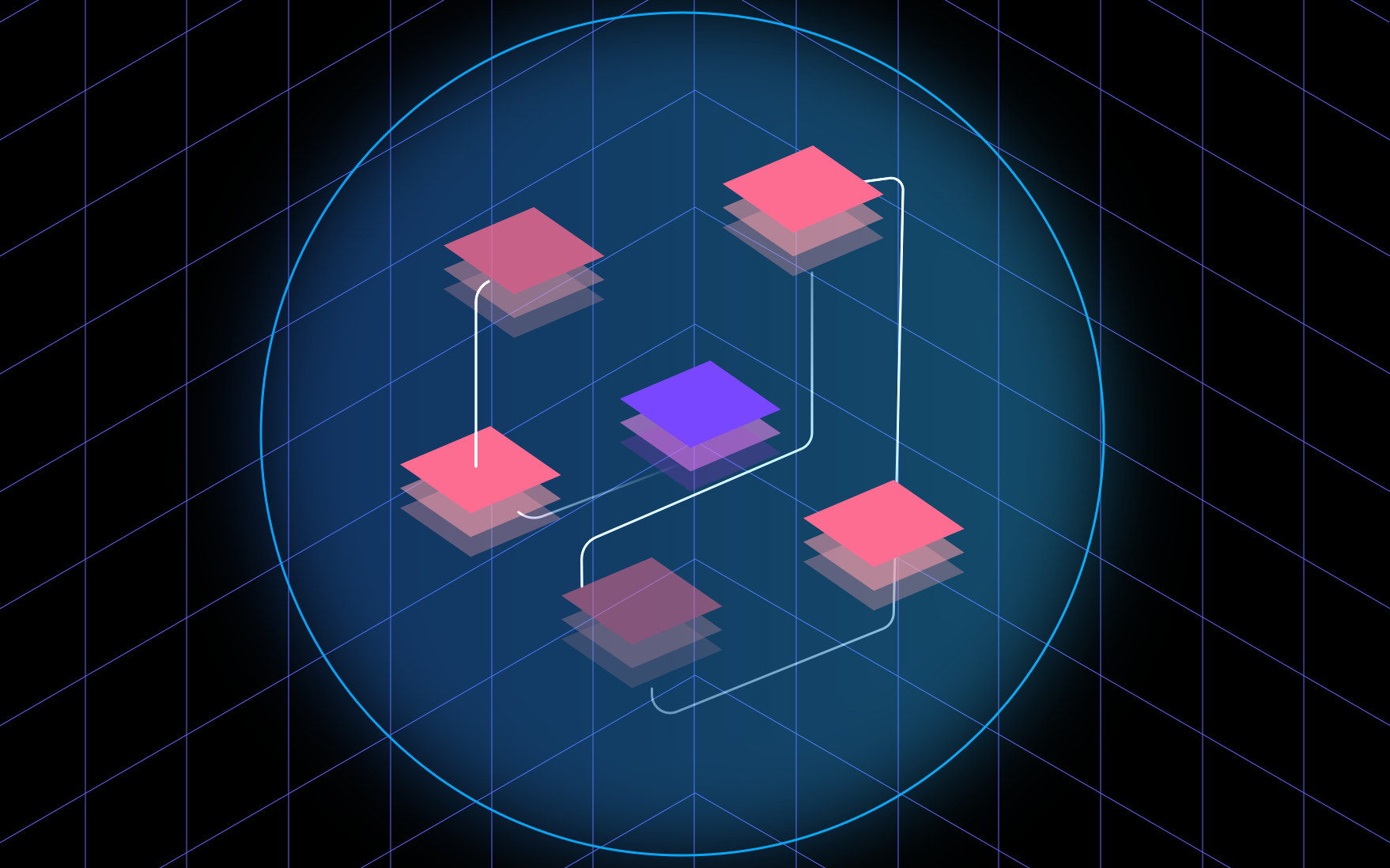-How Much Training Data Do I Need?

Do I have enough data to train a useful model? This is an important question to consider when you are training and evaluating a machine learning algorithm. However, you should consider several other questions, such as: Is my data sufficiently balanced? Does that data cover the full spectrum of the problem that I am looking to solve? To find the answers, you can use heuristic methods and, of course, a few lines of code.

In the realm of machine learning, you can see how much influence something has on the learning process by examining the learning curve. With this, you can plot how much a model improves by adding more data to its training and then checking its score.

Below, we will show you a simple example of how you can use Python to read a learning curve using a classification model with a dataset containing three balanced classes. The standard rule-of-thumb is to use 70% of the available data for training and 30% for testing. First, we’ll use this function to plot the learning curve:

<pre><code class="python">import numpy as np
import matplotlib.pyplot as plt
from sklearn.datasets import fetch_openml
from sklearn.dummy import DummyClassifier
from sklearn.model_selection import learning_curve
from sklearn.linear_model import LogisticRegression
from sklearn.model_selection import train_test_split

%matplotlib inline
rseed = 42
train_sizes = np.linspace(0.1, 1.0, 10)
data, target = fetch_openml(data_id=60, return_X_y = True)

X_train, X_test, y_train, y_test = train_test_split(data, target, test_size=0.3, stratify=target, random_state=rseed)

# We'll train a dummy model, and then plot the median & SD of train + test

dmodel = DummyClassifier(strategy='most_frequent')
_, dtrain_scores, dtest_scores = learning_curve(estimator=dmodel, X=X_train, y=y_train, cv=5, train_sizes=train_sizes)

fig, ax = plt.subplots(1)
ax.plot(train_sizes, np.mean(dtrain_scores, axis=1), color='red', marker='o', label='Dummy Train acc')
upper_std = np.mean(dtrain_scores, axis=1) + np.std(dtrain_scores, axis=1)
lower_std = np.mean(dtrain_scores, axis=1) - np.std(dtrain_scores, axis=1)
ax.fill_between(train_sizes, upper_std, lower_std, alpha=0.15, color='red')

ax.plot(train_sizes, np.mean(dtest_scores, axis=1), color='green', marker='o', label='Dummy Test acc')
upper_std = np.mean(dtest_scores, axis=1) + np.std(dtest_scores, axis=1)
lower_std = np.mean(dtest_scores, axis=1) - np.std(dtest_scores, axis=1)
ax.fill_between(train_sizes, upper_std, lower_std, alpha=0.15, color='green')

ax.set_title('Learning curve')
plt.grid()
plt.legend()</code></pre>

When we test the performance of our dummy model, we see that it doesn’t score so well (around 33%), and it doesn’t improve if we increase the number of training samples. Here, we have an underfitting model, and we need to either improve the model or increase the number of features in order to get a better score. In our specific case a simple change in the algorithm selected improves the score notably. Remember that there is no silver bullet in ML and you should check several possible algorithms and hyperparameters to find the proper way to solve a specific problem.

On the other hand, a model that performs well with a limited number of samples can be improved by increasing the size of the testing set. Eventually, though, we’ll get to the point where adding more samples will be far less valuable than acquiring new ones. Here, we will enter the danger zone of an overfitted model. We can make a better model with the same dataset as follows (notice that the gain from the new samples is marginal):

Be sure that you don’t include all available samples of a particular class in the training dataset, and keep in mind that all models are overfitted to some extent. A significantly overfitted model, though, will be of no use in the real world, and the patterns that you predict might not actually be there.

How Do You Get More Data?

It’s not unusual to get a dataset that is imbalanced, meaning that one or more classes are clearly underrepresented. This can be discovered with a simple EDA such as the Wine Quality Data Set, which includes seven classes with the following distribution:

Among other techniques, we can get more data by oversampling the under-represented classes. One simple solution is to use the imbalanced-learn package, which provides several algorithms for increasing the quantity of training data. In a real-world scenario it would make more sense to use an automated solution to label your data like Watchful, this kind of tool would accelerate and improve your pipeline as it includes advanced features like probabilistic labels. For our example, we can oversample the smallest class of our dataset as follows:

<pre><code class="python">from imblearn.over_sampling import RandomOverSampler
ros = RandomOverSampler(random_state=42, sampling_strategy='minority')
X_resampled, y_resampled = ros.fit_resample(data, target)</code></pre>

Now, the distribution of the classes looks different. Imbalanced-learn also includes algorithms for creating synthetic examples by interpolating data from the minority classes. It’s worth noting that although this would be a cheaper way to increase your available data, you must make sure that the new synthetic data makes sense in the context of your particular problem.

Speaking of interpolation, many algorithms perform well with tasks that were predicted during training (interpolation), but fail to predict others. For example, when we train a neural network to predict a sigmoid function, it fails to predict values outside of the training space:

<pre><code class="python">flow.keras.models import Sequential
from tensorflow.keras.layers import Dense, Activation

def sigmoid(x):
return 1/(1 + np.exp(-x))

data = np.linspace(-10, 10, 100)
target = sigmoid(data)

model = Sequential()
model.fit(data,target, epochs=1000, verbose=0)

data = np.arange(-30.0, 30.0, 1.0)
pred = model.predict(data)
target = sigmoid(data)

plt.plot(data, target, color='green', label='Sigmoid')
plt.plot(data, pred, color='red', label='NN')
plt.title('Sigmoid vs prediction')
plt.xlabel("x")
plt.grid()
plt.legend(loc='lower right')
plt.show()</code></pre>

In such cases, one solution might be to generate samples between the minimum and maximum of all features of the problem, creating a kind of hypercube that would enable the model to learn from all possible values in the problem space.

Conclusion

Of course, real problems will be more complex than the simple example that we used here, but following this basic strategy will help you see if you have enough data to work with, if your dataset is properly balanced, and if it accounts for all possible scenarios that you need to consider before making a generalization. You can find all of the code that we used in this article at Github.